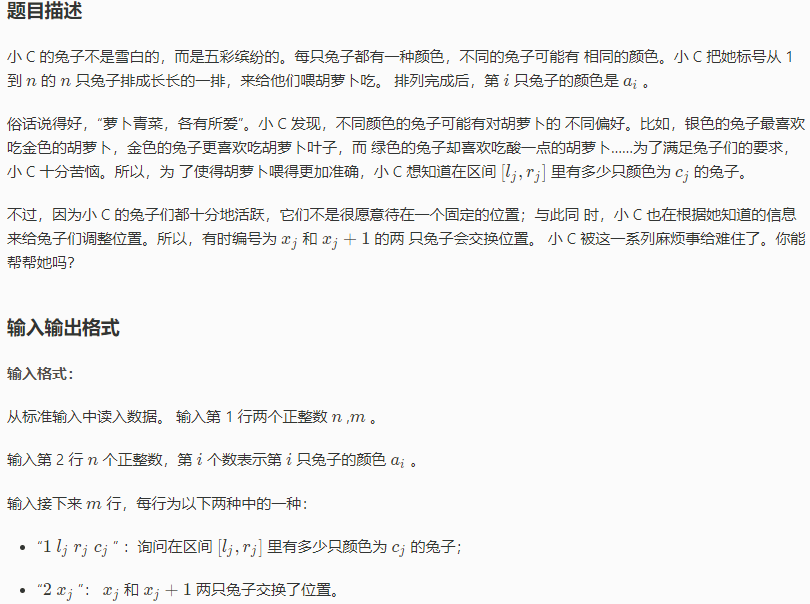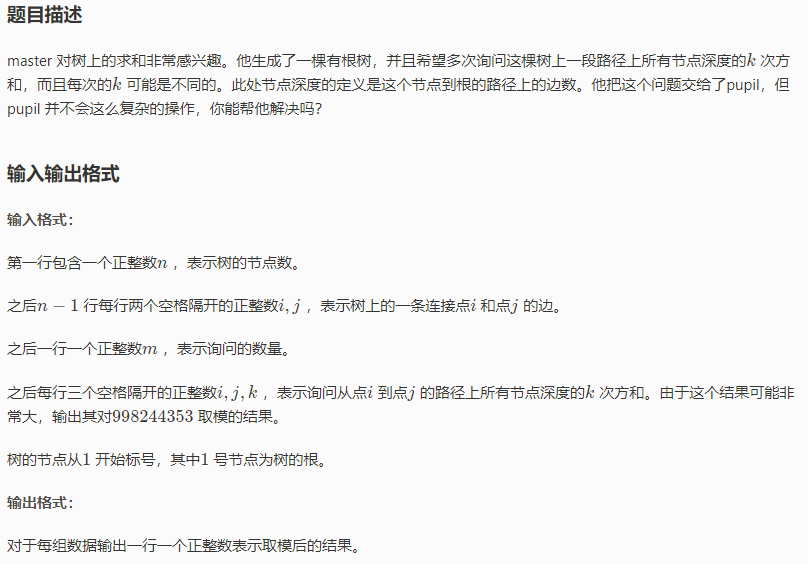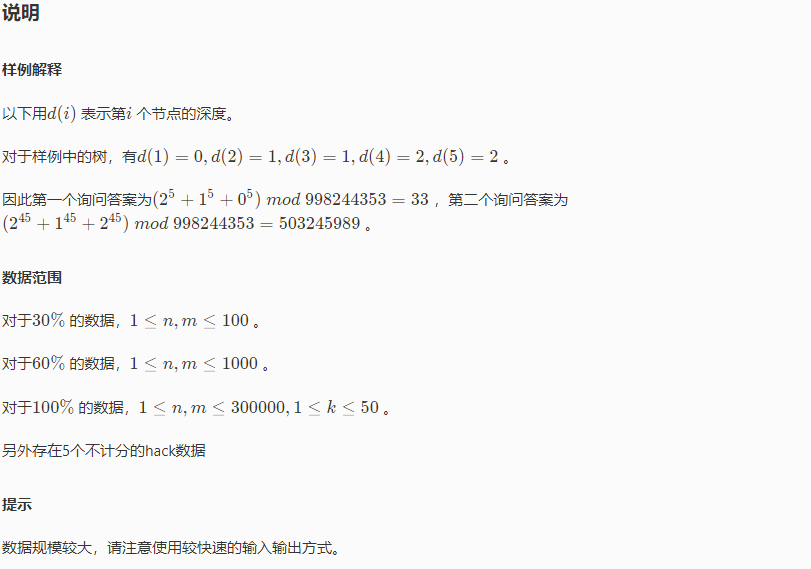2020牛客多校第二场H-Happy Triangle$$对查询的边x，分为两种情况$$

$$第一种，x为最大边，只需要找离x最近且比x小的两条边判断a+b>x就够了，此处可用multiset$$

$$第二种，x不是最大边，那么需要找到一个b，使得b \geq x，b-a<x$$[……]

[LOJ10009]钓鱼$$枚举每一个湖k作为终止点，期间的钓鱼次数则为\frac{60H+5\sum_{i=1}^{k-1} T_{i}}{5}$$

$$要使钓到数量最多，则需要保证每一次钓到的鱼最多，用优先队列维护$$

\$latex 要注意\frac{60H+5\sum_{[……]

[LOJ144]DFS 序 1$$先dfs一遍，idx[i]记录结点i的dfs序，last[i]记录结点i的子树的最后一个结点的dfs序$$

$$用树状数组维护，答案为query(last[a])-query(idx[a]-1)$$

[crayon-61e6ec7709dc6[……]

【模板】左偏树（可并堆）

题目描述

0：后接两个整数(x，y)，代表询问从x到y的路径上的点的权值的xor和。保证x到y是联通的。

1：后接两个整数(x，y)，代表连接x到y，若x到y已经[……]

【模板】二逼平衡树（树套树）

题目描述

1. 查询k在区间内的排名
2. 查询区间内排名为k的值
3. 修改某一位值上的数值
4. 查询k在区间内的前驱(前驱定义为严格小于x，且最大的数，若不存在输出-2147483647)
5. 查询k在[……]

数颜色输入输出样例

[crayon-61e6ec770c8[……]

[BJOI2018]求和输入输出样例